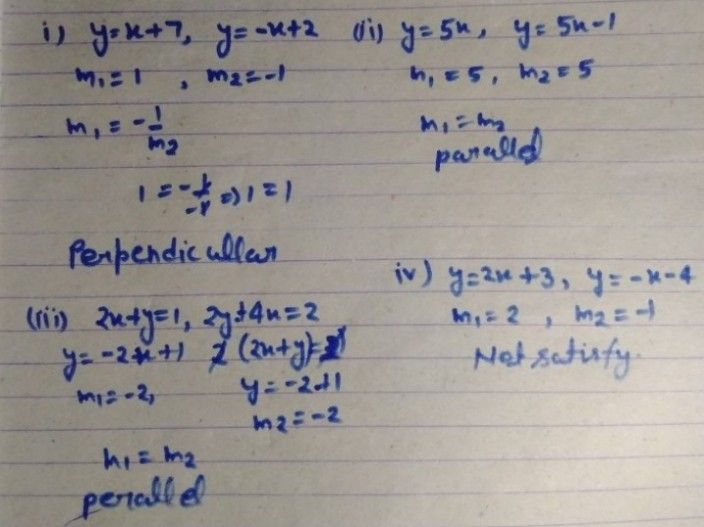Symbol
Problemparallel, If each line in the system has the same slope and the $samcy-lntg$ the (d) lines are coincident. The lines are on top of each other. y-intercept, Learning Task 2. Without graphing, tell whether the graphs of the system of equations are parallel, perpendicular, intersecting or coinciding. $D01tinyoa\pi$ notebook. $1.y=x+7$ and $y=-x+2$ 3. 2x + y = 1 and $2y+4x=2$ 2.Y = 5x $and$ $y=5x-1$ 4. $y=2x+3$ and y = -x - 4 E Learning Task 3. Graph the system of equations 1. $y=x+5$ $andy=5x+5$ 2. $x-3y=-12$ and $4x+6y=-12$ $3.$ $x+2y=-5$ and $-2x+y=3$ $4.$ $y=3x-2$ and $y=-x-6$ 5. $2x-3y=-2$ $and$ $4x+y=24$ PIVOT 4A $ACALABARZON$
7th-9th grade
Other
Search count: 123
SolutionQanda teacher - RASHMIKAplease evaluate ans with gift coins
please